# Excel array formulas, functions and constants - examples and guidelines

In this tutorial, you will learn what an Excel array formula is, how to enter it correctly in your worksheets, and how to use array constants and array functions.

Array formulas in Excel are an extremely powerful tool and one of the most difficult to master. A single array formula can perform multiple calculations and replace thousands of usual formulas. And still, 90% of users have never used array functions in their worksheets simply because they are scared to start learning them.

Indeed, array formulas one of the most confusing Excel features to learn. The aim of this tutorial is to make the learning curve as easy and smooth as possible.

## What is an array in Excel?

Before we start on array functions and formulas, let's figure out what the term "array" means. Essentially, an array is a collection of items. The items can be text or numbers and they can reside in a single row or column, or in multiple rows and columns.

For example, if you put your weekly grocery list into an Excel array format, it would look like:

{"Milk", "Eggs", "Butter", "Corn flakes"}

Then, if you select cells A1 through D1, enter the above array preceded by an equal sign (=) in the formula bar and press CTRL + SHIFT + ENTER, you will get the following result: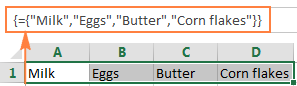What you have just done is create a one-dimensional horizontal array. Nothing dreadful so far, right?

## What is an array formula in Excel?

The difference between an array formula and a regular formula is that an array formula processes several values instead of just one. In other words, an array formula in Excel evaluates all individual values in an array and performs multiple calculations on one or several items according to the conditions expressed in the formula.

Not only can an array formula deal with several values simultaneously, it can also return several values at a time. So, the results returned by an array formula is also an array.

Array formulas are available in all versions of Excel 2019, Excel 2016, Excel 2013, Excel 2010, Excel 2007 and lower.

And now, it seems to be the right time for you to create your first array formula.

#### Simple example of Excel array formula

Suppose you have some items in column B, their prices in column C, and you want to calculate the grand total of all sales.

Of course, nothing prevents you from calculating subtotals in each row first with something as simple as `=B2*C2` and then sum those values: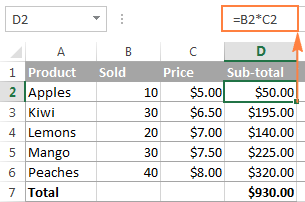However, an array formula can spare you those extra key strokes since it gets Excel to store intermediate results in memory rather than in an additional column. So, all it takes is a single array formula and 2 quick steps:

1. Select an empty cell and enter the following formula in it:

`=SUM(B2:B6*C2:C6)`

2. Press the keyboard shortcut CTRL + SHIFT + ENTER to complete the array formula.

Once you do this, Microsoft Excel surrounds the formula with {curly braces}, which is a visual indication of an array formula.

What the formula does is multiply the values in each individual row of the specified array (cells B2 through C6), add the sub-totals together, and output the grand total: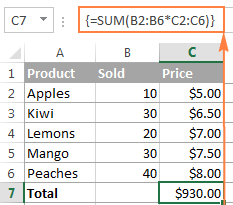This simple example shows how powerful an array formula can be. When working with hundreds and thousands of rows of data, just think how much time you can save by entering one array formula in a single cell.

#### Why use array formulas in Excel?

Excel array formulas are the handiest tool to perform sophisticated calculations and do complex tasks. A single array formula can replace literally hundreds of usual formulas. Array formulas are very good for tasks such as:

## How to enter array formula in Excel (Ctrl + Shift + Enter)

As you already know, the combination of the 3 keys CTRL + SHIFT + ENTER is a magic touch that turns a regular formula into an array formula.

When entering an array formula in Excel, there are 4 important things to keep in mind:

1. Once you've finished typing the formula and simultaneously pressed the keys CTRL SHIFT ENTER, Excel automatically encloses the formula between {curly braces}. When you select such a cell(s), you can see the braces in the formula bar, which gives you a clue that an array formula is in there.
2. Manually typing the braces around a formula won't work. You must press the Ctrl+Shift+Enter shortcut to complete an array formula.
3. Every time you edit an array formula, the braces disappear and you must press Ctrl+Shift+Enter again to save the changes.
4. If you forget to press Ctrl+Shift+Enter, your formula will behave like a usual formula and process only the first value(s) in the specified array(s).

Because all Excel array formulas require pressing Ctrl + Shift + Enter, they are sometimes called CSE formulas.

## Use the F9 key to evaluate portions of an array formula

When working with array formulas in Excel, you can observe how they calculate and store their items (internal arrays) to display the final result you see in a cell. To do this, select one or several arguments within a function's parentheses, and then press the F9 key. To exit the formula evaluation mode, press the Esc key.

In the above example, to see the sub-totals of all products, you select B2:B6*C2:C6, press F9 and get the following result.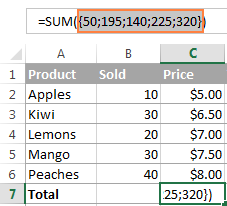Note. Please pay attention that you must select some part of the formula prior to pressing F9, otherwise the F9 key will simply replace your formula with the calculated value(s).

## Single-cell and multi-cell array formulas in Excel

Excel array formula can return a result in a single cell or in multiple cells. An array formula entered in a range of cells is called a multi-cell formula. An array formula residing in a single cell is called a single-cell formula.

There exist a few Excel array functions that are designed to return multi-cell arrays, for example TRANSPOSE, TREND, FREQUENCY, LINEST, etc.

Other functions, such as SUM, AVERAGE, AGGREGATE, MAX, MIN, can calculate array expressions when entered into a single cell by using Ctrl + Shift + Enter.

The following examples demonstrate how to use a single-cell and multi-cell array formula.

#### Example 1. A single-cell array formula

Suppose you have two columns listing the number of items sold in 2 different months, say columns B and C, and you want to find the maximum sales increase.

Normally, you would add an additional column, say column D, that calculates the sales change for each product using a formula like `=C2-B2`, and then find the maximum value in that additional column `=MAX(D:D)`.

An array formula does not need an additional column since it perfectly stores intermediate results in memory. So, you just enter the following formula and press Ctrl + Shift + Enter:

`=MAX(C2:C6-B2:B6)`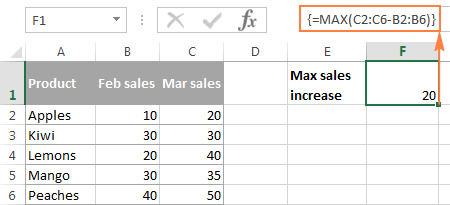#### Example 2. A multi-cell array formula in Excel

In the previous SUM example, suppose you have to pay 10% tax from each sale and you want to calculate the tax amount for each product with one formula.

Select the range of cell in some blank column, say D2:D6, and enter the following formula in the formula bar:

`=B2:B6 * C2:C6 * 0.1`

Once you press Ctrl + Shift + Enter, Excel will place an instance of your array formula in each cell of the selected range, and you will get the following result: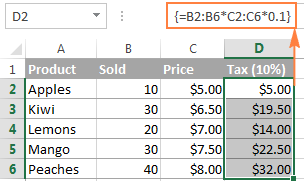#### Example 3. Using an Excel array function to return a multi-cell array

As already mentioned, Microsoft Excel provides a few so called "array functions" that are specially designed to work with multi-cell arrays. TRANSPOSE is one of such functions and we are going to utilize it to transpose the above table, i.e. convert rows to columns.

1. Select an empty range of cells where you want to output the transposed table. Since we are converting rows to columns, be sure to select the same number of rows and columns as your source table has columns and rows, respectively. In this example, we are selecting 6 columns and 4 rows.
2. Press F2 to enter the edit mode.
3. Enter the array function `=TRANSPOSE(array)` and press Ctrl + Shift + Enter. In our example, the formula is `=TRANSPOSE(\$A\$1:\$D\$6)`.

The result is going to look similar to this: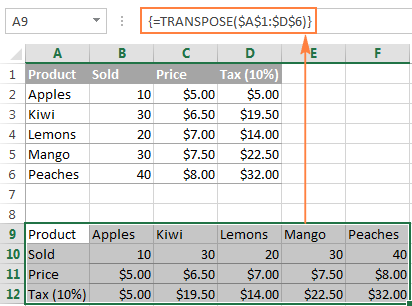This is how you use an array function in Excel. To learn the nuts and bolts of TRANSPOSE, please check out this tutorial: How to transpose in Excel - convert rows to columns.

#### How to work with multi-cell array formulas

When working with multi-cell array formulas in Excel, be sure to follow these rules to get the correct results:

1. Select the range of cells where you want to output the results before entering the formula.
2. To delete a multi-cell array formula, either select all the cells containing it and press DELETE, or select the entire formula in the formula bar, press DELETE, and then press Ctrl + Shift + Enter.
3. You cannot edit or move the contents of an individual cell in an array formula, nor can you insert new cells into or delete existing cells from a multi-cell array formula. Whenever you try doing this, Microsoft Excel will throw the warning "You cannot change part of an array".
4. To shrink an array formula, i.e. to apply it to fewer cells, you need to delete the existing formula first and then enter a new one.
5. To expand an array formula, i.e. apply it to more cells, select all cells containing the current formula plus empty cells where you want to have it, press F2 to switch to the edit mode, adjust the references in the formula and press Ctrl + Shift + Enter to update it.
6. You cannot use multi-cell array formulas in Excel tables.
7. You should enter a multi-cell array formula in a range of cells of the same size as the resulting array returned by the formula. If your Excel array formula produces an array larger than the selected range, the excess values won't appear on the worksheet. If an array returned by the formula is smaller than the selected range, #N/A errors will appear in extra cells.

If your formula may return an array with a variable number of elements, enter it in a range equal to or larger than the maximum array returned by the formula and wrap your formula in the IFERROR function, as demonstrated in this example.

## Excel array constants

In Microsoft Excel, an array constant is simply a set of static values. These values never change when you copy a formula to other cells or values.

You already saw an example of an array constant created from a grocery list in the very beginning of this tutorial. Now, let's see what other array types exist and how you create them.

There exist 3 types of array constants:

#### 1. Horizontal array constant

A horizontal array constant resides in a row. To create a row array constant, type the values separated by commas and enclose then in braces, for example {1,2,3,4}.

Note. When creating an array constant, you should type the opening and closing braces manually.

To enter a horizontal array in a spreadsheet, select the corresponding number of blank cells in a row, type the formula `={1,2,3,4}` in the formula bar, and press Ctrl + Shift + Enter. The result will be similar to this: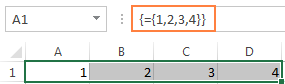As you see in the screenshot, Excel wraps an array constant in another set of braces, exactly like it does when you are entering an array formula.

#### 2. Vertical array constant

A vertical array constant resides in a column. You create it in the same way as a horizontal array with the only difference that you delimit the items with semicolons, for example:

`={11; 22; 33; 44}`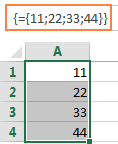#### 3. Two-dimensional array constant

To create a two-dimensional array, you separate each row by a semicolon and each column of data by a comma.

`={"a", "b", "c"; 1, 2, 3}`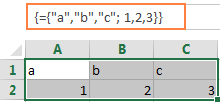### Working with Excel array constants

Array constants are one of the cornerstones of an Excel array formula. The following information and tips might help you use them in the most efficient way.

1. Elements of an array constant

An array constant can contain numbers, text values, Booleans (TRUE and FALSE) and error values, separated by commas or semicolons.

You can enter a numerical value as an integer, decimal, or in scientific notation. If you use text values, they should be surrounded in double quotes (") like in any Excel formula.

An array constant cannot include other arrays, cell references, ranges, dates, defined names, formulas, or functions.

2. Naming array constants

To make an array constant easier to use, give it a name:

• Switch to the Formulas tab > Defined Names group and click Define Name. Alternatively, press Ctrl + F3 and click New.
• Type the name in the Name
• In the Refers to box, enter the items of your array constant surrounded in braces with the preceding equality sign (=). For example:

`={"Su", "Mo", "Tu", "We", "Th", "Fr", "Sa"}`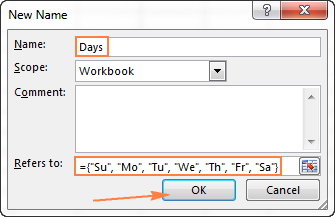• Click OK to save your named array and close the window.

To enter the named array constant in a sheet, select as many cells in a row or column as there are items in your array, type the array's name in the formula bar preceded with the = sign and press Ctrl + Shift + Enter.

The result should resemble this: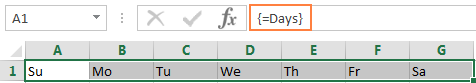3. Preventing errors

If your array constant does not work correctly, check for the following problems:

• Delimit the elements of your array constant with the proper character - comma in horizontal array constants and semicolon in vertical ones.
• Selected a range of cells that exactly matches the number of items in your array constant. If you select more cells, each extra cell will have the #N/A error. If you select fewer cells, only a part of the array will be inserted.

### Using array constants in Excel formulas

Now that you are familiar with the concept of array constants, let's see how you can use arrays informulas to solve your practical tasks.

#### Example 1. Sum N largest / smallest numbers in a range

You start by creating a vertical array constant containing as many numbers as you want to sum. For example, if you want to add up 3 smallest or largest numbers in a range, the array constant is {1,2,3}.

Then, you take either LARGE or SMALL function, specify entire range of cells in the first parameter and include the array constant in the second. Finally, embed it in the SUM function, like this:

Sum the largest 3 numbers: `=SUM(LARGE(range, {1,2,3}))`

Sum the smallest 3 numbers: `=SUM(SMALL(range, {1,2,3}))`

Don't forget to press Ctrl + Shift + Enter since you are entering an array formula, and you will get the following result: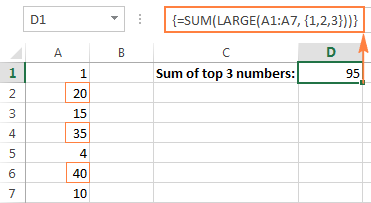In a similar fashion, you can calculate the average of N smallest or largest values in a range:

Average of the top 3 numbers: `=AVERAGE(LARGE(range, {1,2,3}))`

Average of the bottom 3 numbers: `=AVERAGE(SMALL(range, {1,2,3}))`

#### Example 2. Array formula to count cells with multiple conditions

Suppose, you have a list of orders and you want to know how many times a given seller has sold given products.

The easiest way would be using a COUNTIFS formula with multiple conditions. However, if you want to include many products, your COUNTIFS formula may grow too big in size. To make it more compact, you can use COUNTIFS together with SUM and include an array constant in one or several arguments, for example:

`=SUM(COUNTIFS(range1, "criteria1", range2, {"criteria1", "criteria2"}))`

The real formula may look as follows:

`=SUM(COUNTIFS(B2:B9, "sally", C2:C9, {"apples", "lemons"}))`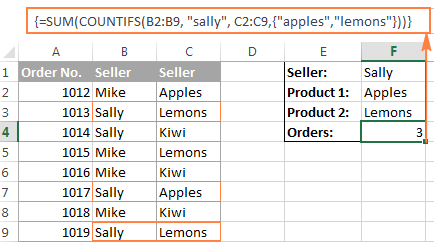Our sample array consists of only two elements since the goal is to demonstrate the approach. In your real array formulas, you may include as many elements as your business logic requires, provided that the total length of the formula does not exceed 8,192 characters in Excel 2019 - 2007 (1,024 characters in Excel 2003 and lower) and your computer is powerful enough to process large arrays. Please see the limitations of array formulas for more details.

And here is an advanced array formula example that finds the sum of all matching values in a table: SUM and VLOOKUP with an array constant.

## AND and OR operators in Excel array formulas

An array operator tells the formula how you want to process the arrays - using AND or OR logic.

• AND operator is the asterisk (*) which is the multiplication symbol. It instructs Excel to return TRUE if ALL of the conditions evaluate to TRUE.
• OR operator is the plus sign (+). It returns TRUE if ANY of the conditions in a given expression evaluates to TRUE.

#### Array formula with the AND operator

In this example, we find the sum of sales where the sales person is Mike AND the product is Apples:

`=SUM((A2:A9="Mike") * (B2:B9="Apples") * (C2:C9))`

Or

`=SUM(IF(((A2:A9="Mike") * (B2:B9="Apples")), (C2:C9)))`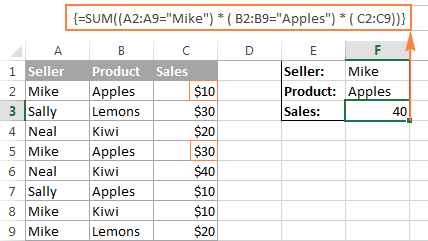Technically, this formula multiplies the elements of the three arrays in the same positions. The first two arrays are represented by TRUE and FALSE values which are the results of comparing A2:A9 to Mike" and B2:B9 to "Apples". The third array contains the sales numbers from the range C2:C9. Like any math operation, multiplication converts TRUE and FALSE to 1 and 0, respectively. And because multiplying by 0 always gives zero, the resulting array has 0 when either or both conditions are not met. If both conditions are met, the corresponding element from the third array gets into the final array (e.g. 1*1*C2 = 10). So, the result of multiplication is this array: {10;0;0;30;0;0;0;0}. Finally, the SUM function adds up the array's elements and return a result of 40.

#### Excel array formula with the OR operator

The following array formula with the OR operator (+) adds up all sales where the sales person is Mike OR product is Apples:

`=SUM(IF(((A2:A9="Mike") + (B2:B9="Apples")), (C2:C9)))`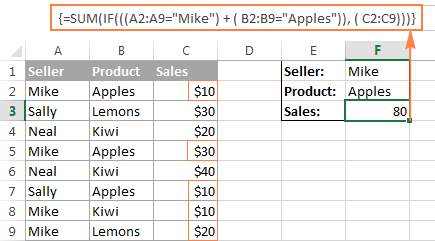In this formula, you add up the elements of the first two arrays (which are the conditions you want to test), and get TRUE (>0) if at least one condition evaluates to TRUE; FALSE (0) when all the conditions evaluates to FALSE. Then, IF checks if the result of addition is greater than 0, and if it is, SUM adds up a corresponding element of the third array (C2:C9).

Tip. In modern versions of Excel, there is no need to use an array formula for this kind of tasks - a simple SUMIFS formula handles them perfectly. Nevertheless, the AND and OR operators in array formulas may prove helpful in more complex scenarios, let alone a very good gymnastics of mind : )

## Double unary operator in Excel array formulas

If you've ever worked with array formulas in Excel, chances are you came across a few ones containing a double dash (--) and you may have wondered what it was used for.

A double dash, which is technically called the double unary operator, is used to convert non-numeric Boolean values (TRUE / FALSE) returned by some expressions into 1 and 0 that an array function can understand.

The following example will hopefully make things easier to understand. Suppose you have a list of dates in column A and you want to know how many dates occur in January, regardless of the year.

The following formula will work a treat:

`=SUM(--(MONTH(A2:A10)=1))`

Since this is an Excel array formula, remember to press Ctrl + Shift + Enter to complete it.

If you are interested in some other month, replace 1 with a corresponding number. For example, 2 stands for February, 3 means March, and so on. To make the formula more flexible, you can specify the month number in some cell, like demonstrated in the screenshot: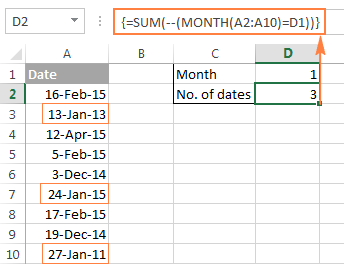And now, let's analyze how this array formula works. The MONTH function returns the month of each date in cells A2 through A10 represented by a serial number, which producing the array {2;1;4;2;12;1;2;12;1}.

After that, each element of the array is compared to the value in cell D1, which is number 1 in this example. The result of this comparison is an array of Boolean values TRUE and FALSE. As you remember, you can select a certain portion of an array formula and press F9 to see what that part equates to: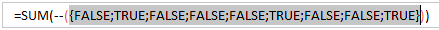Finally, you have to convert these Boolean values to 1's and 0's that the SUM function can understand. And this is what the double unary operator is needed for. The first unary coerces TRUE/FALSE to -1/0, respectively. The second unary negates the values, i.e. reverses the sign, turning them into +1 and 0, which most of Excel functions can understand and work with. If you remove the double unary from the above formula, it won't work.

I am hopeful this short tutorial has proved helpful on your road to mastering Excel array formulas. Next week, we are going to continue with Excel arrays by focusing on advanced formula examples. Please stay tuned and thank you for reading!

## You may also be interested inWar in Ukraine. Here's what Ablebits is doing to make sure our team and projects are safe.